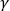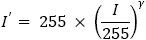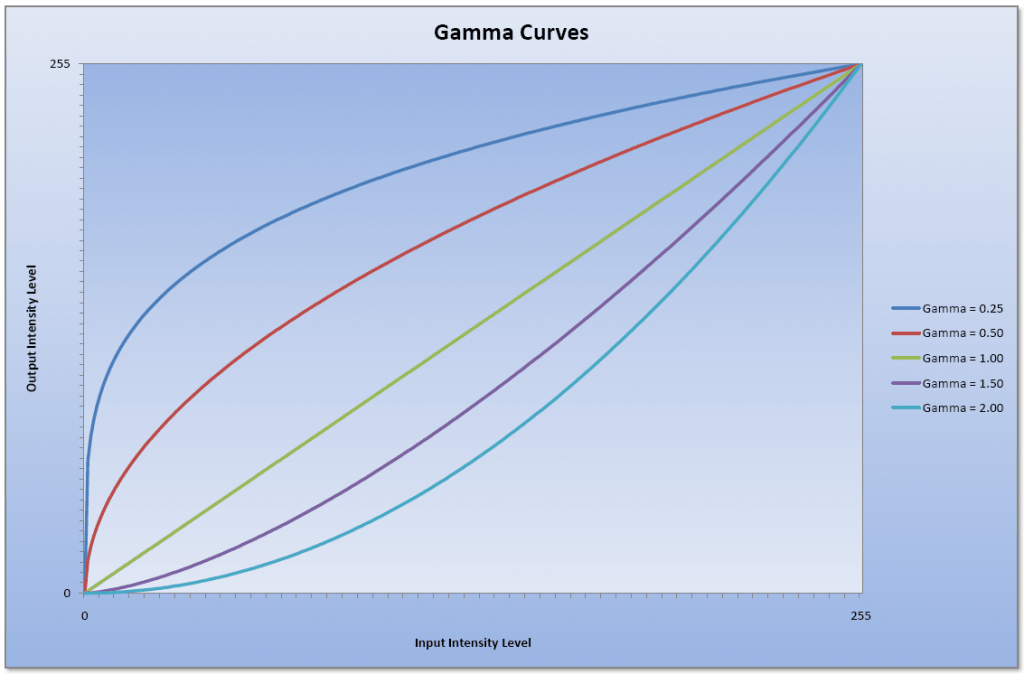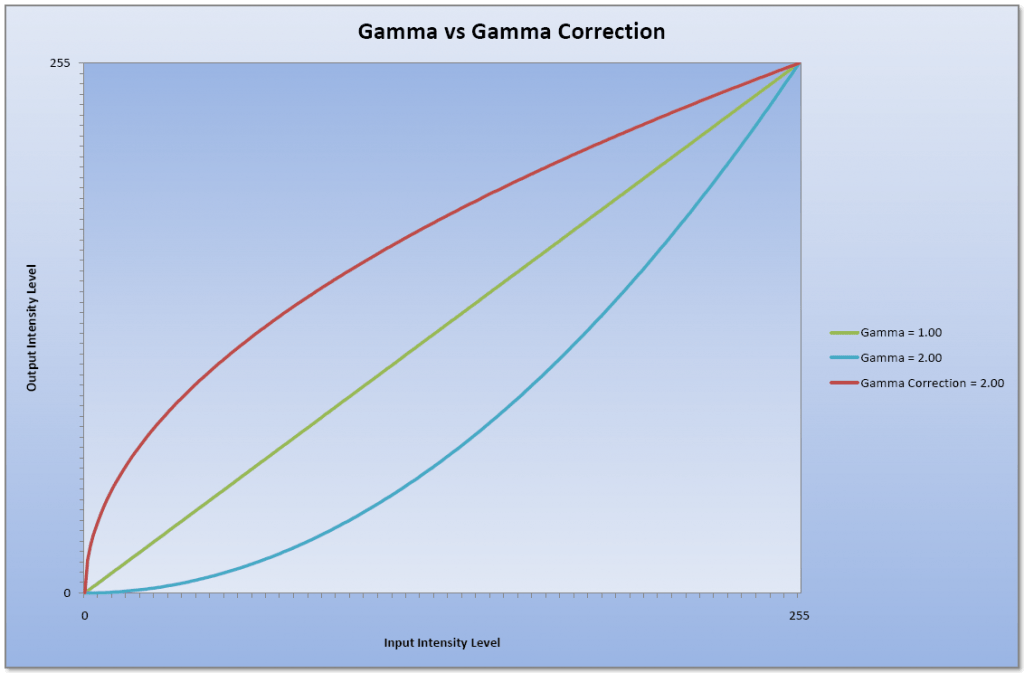# Image Processing Algorithms Part 6: Gamma Correction

This article was originally published in issue 57 of The Crypt Mag

Gamma, represented by the Greek letter, can be described as the relationship between an input and the resulting output. For the scope of this article the input will be the RGB intensity values of an image.

The relationship in this case between the input and output is that the output is proportional to the input raised to the power of gamma. The formula for calculating the resulting output is as follows:To illustrate, here are a few gamma curves:Various Gamma Curves
(click image to enlarge)

Note that with a gamma of 1 the input equals the output producing a straight line.

For calculating gamma correction the input value is raised to the power of the inverse of gamma. The formula for this is as follows:The following graph shows a comparison between the gamma curve and the gamma correction curve:Gamma versus Gamma Correction
(click image to enlarge)

In pseudo-code performing gamma correction would go something like this:

```   gammaCorrection = 1 / gamma
colour = GetPixelColour(x, y)
newRed   = 255 * (Red(colour)   / 255) ^ gammaCorrection
newGreen = 255 * (Green(colour) / 255) ^ gammaCorrection
newBlue  = 255 * (Blue(colour)  / 255) ^ gammaCorrection
PutPixelColour(x, y) = RGB(newRed, newGreen, newBlue)
```

The range of values used for gamma will depend on the application, but personally I tend to use a range of 0.01 to 7.99.

Here we have the ‘Lena’ and ‘Mandrill’ images which have had the gamma corrected using a gamma of 0.25 and 2.00:‘Lena’ image with gamma of 0.25 (left) and 2.00 (right)
(click images to enlarge)‘Mandrill’ image with gamma of 0.25 (left) and 2.00 (right)
(click images to enlarge)

There have been a few people that have had trouble getting this algorithm to work properly. The following tip has been provided by Felix in the comments below, but I have reiterated here:

The algorithm works great. A notice for those who code in static type languages, all variables used in the formula must be double type (even if they contain integers), and all integer constants (such as 255) must be written in double type (255.0).

Thanks for the tip Felix! 🙂

## 16 thoughts on “Image Processing Algorithms Part 6: Gamma Correction”

1.rat says:

Hey thanks for the article, finding this series really useful.

However whenever I run this, my result it a black image with roughly 5 random pixels visible no matter what value I input as the Gamma. I’ve tried narrowing it down line by line but can’t seem to figure out why.

I’ve set the gamma and gammaCorrection variables as float and double yet I achieve the same result either way.

Do you have any idea (from my vague explanation xD) as to why this could be? Cheers

1.Francis Loch says:

Without seeing your code it is difficult for me to guess what is going wrong.

What value(s) of gamma have you tried that produce this effect? Have you tried a gamma of 1.0? Have you tried the brightness adjustment or contrast adjustment tutorials and did they work?

Kind regards,

Francis

1.rat says:

Thanks for your speedy response! Was just about to reply however after going through it line by line one last time I’ve just managed to figure out what I was doing wrong

I was calculating the division “(Red(colour) / 255)” directly on the individual (RGB)pixel values and this was throwing it all off. So I assigned these RGB values to new variables and then performed the gamma calculation and it all works fine.

Thanks for the article and your time to help!

– Jake

1.Francis Loch says:

You’re very welcome! Glad you got it sorted. (^_^)

Kind regards,

Francis

2.Geetha V says:

1.Francis Loch says:

Hi Geetha,

Adaptive gamma correction is not something I have done before. You may want to look at something like this paper which describes a method of achieving this.

Kind regards,

Francis

1.venkatrao says:

by using ur paper i wrote code but iam not getting results .can u give your code

1.Francis Loch says:

My code is more-or-less what is stated in the pseudo-code above. Make sure that you are using a float variable for the gamma correction, otherwise it will not work.

If you are still having problems please feel free to post your implementation of the code here so that I can try and see what is wrong.

Kind regards,

Francis

3.k.radha madhavi says:

could you give me the gamma correction code for grey scale images

1.Francis Loch says:

The code mentioned will also work with greyscale images as the red, green and blue values for each pixel will be the same. You can optimise it though by just taking the intensity value of the greyscale pixel and using something like the following pseudo-code:

```gammaCorrection = 1 / gamma intensity = GetPixelIntensity(x, y) newIntensity = 255 * (Red(intensity) / 255) ^ gammaCorrection PutPixelIntensity(x, y) = newIntensity```

Kind regards,

Francis

4.Lady Gamma says:

Hello!
Your examples show darker images for gamma 1. However, Wikipedia  says it in reverse. Please, do you happen to know what is going on and what is the correct meaning of “gamma”?
Thanks!
 https://en.wikipedia.org/wiki/Gamma_correction

1.Francis Loch says:

The term ‘gamma’ simply refers to the relationship between an input and the resulting output. In our case, as shown above, we end up with a curve known as a gamma curve.

You may have heard of gammas of 1.8 or 2.2 for computer displays. These gamma values refer to how the image would be displayed based on the video information received from the computer. Without correction, the image on the display would appear darker than intended (gamma values greater than 1 will give darker images while gamma values less than 1 will give lighter images).

Gamma correction (which is sometimes, and confusingly, abbreviated to just ‘gamma’) is the process of inverting the original gamma curve to provide an image that is displayed as intended. When I talk about using gamma values of 0.25 and 2 in the article above, these are being applied as gamma correction curves.

Kind regards,

Francis

1.Lady Gamma says:

It does answer my question, thank you!

1.Francis Loch says:

You’re very welcome. 🙂

Kind regards,

Francis

5.Felix says:

The algorithm works great. A notice for those who code in static type languages, all variables used in the formula must be double type (even if they contain integers), and all integer constants (such as 255) must be written in double type (255.0).

1.Francis Loch says:

Thanks for the tip Felix. I’m sure some people will find it useful.

Kind regards,

Francis

This site uses Akismet to reduce spam. Learn how your comment data is processed.